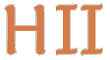# Hilbert II

 English Deutsch
introduction
news
mathematics
QEDEQ
planning
glossary
development
prototype
installation
manual
propositional calculus
predicate calculus
QEDEQ
 module formula QEDEQ text
problems
contact
site map
Prototype / PMII QEDEQ Module / Formula

Here is an first attempt to formalize a formula in the QEDEQ language:

```ALPHABET := {'A', 'B', 'C', .., 'Z', '0', '1', '2', ..,'9', '(', ')'}

WORDS := { (a_1, a_2, a_3, ..., a_n) in alphabet^n, with n a natural
Number or 0}

+ : WORDS -> WORDS
(a_1, ..., a_n), (b_1, ..., b_m) :-> (a_1, ..., a_n, b_1, ..., b_m)

the "empty word" (0-tuple) is a neutral element of this operation.
(ALPHABET, +) has the algebraic structure of an monoid
(half group with neutral element e (the 0-tuple))
as usual, the operation + is extended to work on the
potence set of words (if A subset of WORDS, B subset of WORDS,
then A + B := { a + b | a in A, b in B})

For a shorter writing we write "a + B" iff we mean "{a} + B" (with
a element from WORDS and B a subset from WORDS). Analogous we
write "A + b" iff we mean "A + {b}" (with A a subset from WORDS and
b element from WORDS).

Also for a shorter writing "(", ",", ")" (from tuples) and "+" are
never written. E.g.: "abc" stands for "(a) + (b) + (c)" and
"AB" for "A + B" (with a, b, c elements from ALPHABET and A, B
subsets or elements from WORDS).

We say A is a "sub word" of B, iff there are elements U, V of WORDS
so that B = UAV.

operators on sets of WORDS:
"|" stands for an union (operator has minimal 2 arguments,
infix notation)
A | B := {a element of A or element from B}
"*" stands for an repeated occurrence, the number of these occurrences
could be 0 (one argument, postfix notation)
A* := {e, a, aa, aaa, aaaa, ... with a from A}
"[" "]" stand for an optional occurrence (one argument, pre- and
postfix notation)
[A] := {e} union A

NDIGIT := '1'|'2'|'3'|'4'|'5'|'6'|'7'|'8'|'9'
DIGIT := '0'| NDIGIT
NUMBER := NDIGIT DIGIT*
SUBJECT_VARIABLE := 'VAR(' NUMBER ')'
PREDICATE_VARIABLE :=
'PREDVAR(' NUMBER ',(' SUBJECT_VARIABLE (',' SUBJECT_VARIABLE)* '))'
PROPOSITION_VARIABLE := 'PROP(' NUMBER ')'

ATOMIC_FORMULA := PREDICATE_VARIABLE | PROPOSITION_VARIABLE

We define a function "free" and a function "bound" that maps every
ATOMIC_FORMULA to a subset of SUBJECT_VARIABLE:

free(ATOMIC_FORMULA) -> SUBJECT_VARIABLE

Let F be in PRIME_FORMULA:
free(F) := {S | S is sub word of F and S is in
SUBJECT_VARIABLE}
bound(F) := {}

now we recursively define the subset of WORDS "FORMULA" and extend
the domains of "free" and "bound":

1. every ATOMIC_FORMULA is a FORMULA

2. if F is in FORMULA, then
'NOT(' F ')' is also in FORMULA and we define
free('NOT(' F ')') := free(F)
bound('NOT(' F ')') := bound(F)

3. if F and G are in FORMULA, free(F) and bound(G) have an empty
intersection, bound(F) and free(G) have an empty intersection,
then the following words are also in FORMULA:
'AND(' F ',' G ')'
'OR(' F ',' G ')'
'IMPL(' F ',' G ')'
'EQUI(' F ',' G ')'
Let H be one of these new elements, then we define:
free(H) := free(H) union free(G)
bound(H) := bound(H) union bound(G)

4. if F is in FORMULA, and X is in SUBJECT_VARIABLE with x in
free(X), then the following words are also in FORMULA:
'FORALL(' x ',' f ')'
'EXISTS(' x ',' f ')'
Let h be one of these new formula, then we define:
free(h) := free(f) minus {x}
bound(h) := bound(f) union {x}

```

update 2014-01-20 09:20:48+0100